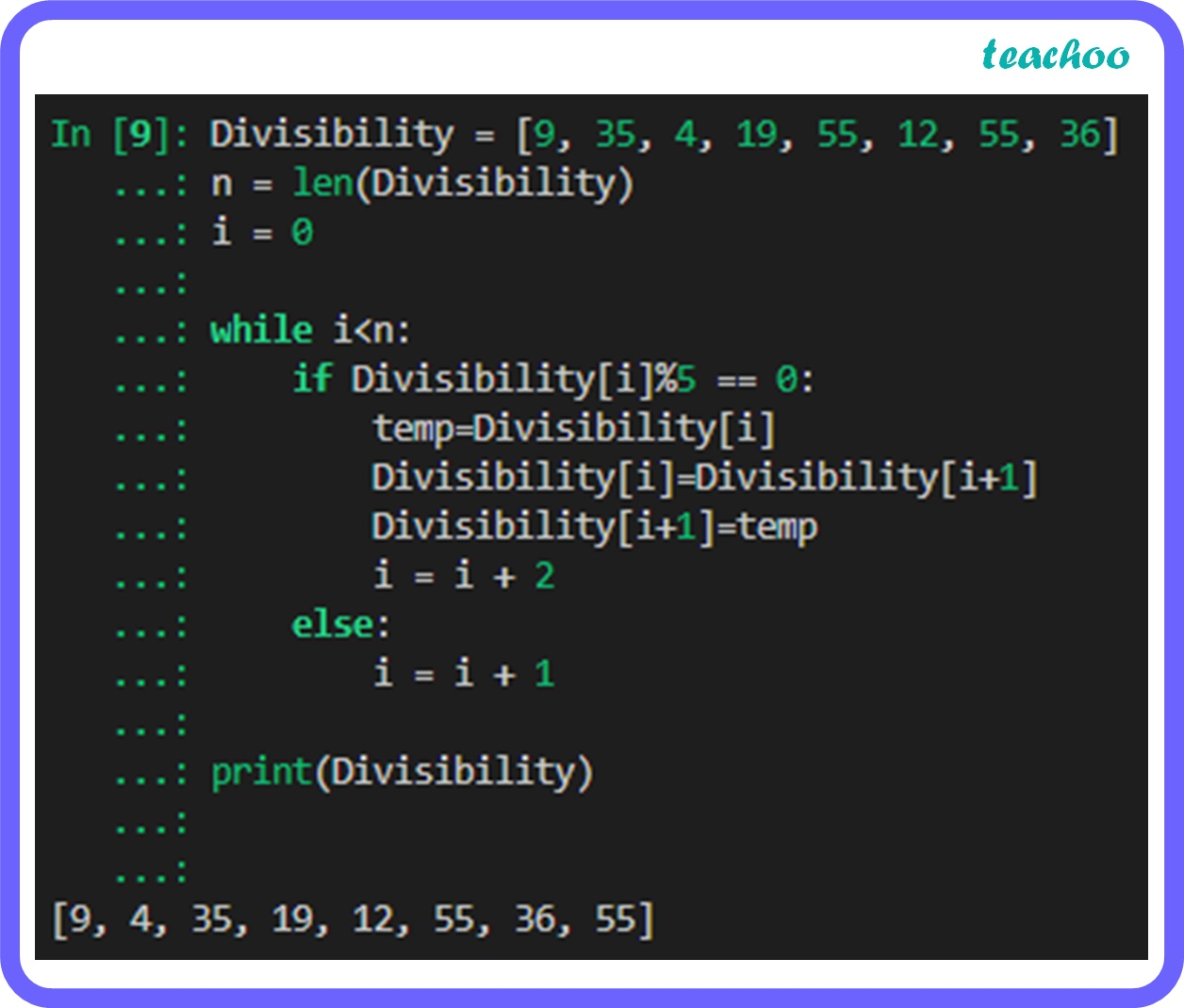Long Answer Type Questions (5 Marks each)

Computer Science - Class 11
Chapter 9 Class 11 - Lists

## 9,4, 35, 19, 12, 55, 36, 55

Code:

``` Divisibility = [ 9 , 35 , 4 , 19 , 55 , 12 , 55 , 36 ]  ```

``` n = len(Divisibility)  ```

``` i = 0   ```

``` while i<n:  ```

```     if Divisibility[i]% 5 == 0 :  ```

```         temp=Divisibility[i]  ```

```         Divisibility[i]=Divisibility[i+ 1 ]  ```

```         Divisibility[i+ 1 ]=temp ```

```         i = i + 2   ```

```     else:  ```

``` i = i + 1   ```

```       ```

``` print(Divisibility) ```Learn in your speed, with individual attention - Teachoo Maths 1-on-1 Class